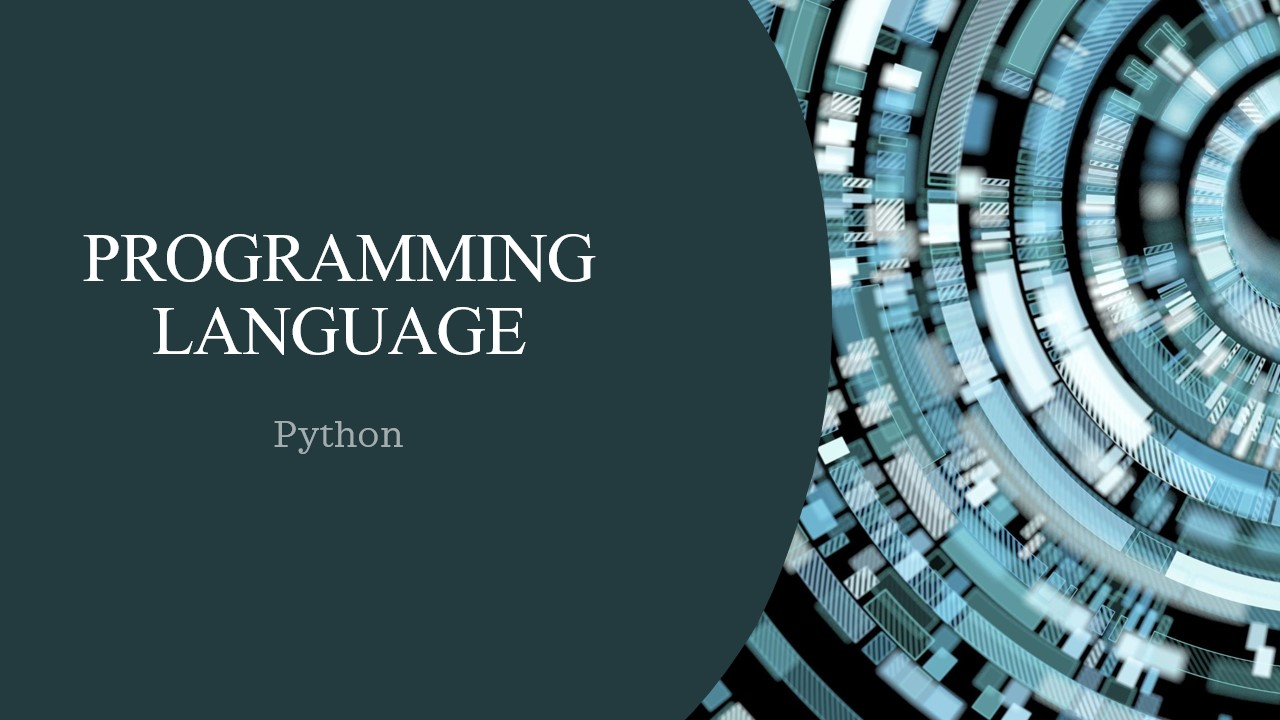/  Technology   /  Convert bytes to a string in Python## Convert bytes to a string in Python

Everything is an object in Python programming, the data types are actually classes and the variables are instances (object) of these classes.

A byte string is an array of bytes with a fixed length. In Python3, byte strings are officially called bytes. It is an immutable sequence of integers in the inclusive range of 0 to 255. The default encoding in Python3 is “UTF-8”.

##### Method 1:

We can convert bytes to a string using the decode() method.

This method works exactly opposite to the encode() method. The decode() method is used to convert from one encoding, in which the argument string is encoded, to the required encoding.

#code for converting bytes to a string using decode()

``` >>h = b'HarryPotter'
>>print(h)  # display input
>>print(type(h))
>>output = h.decode()                # converting
>>print(output) # display output
>>print(type(output))```

Output:

```b'HarryPotter'
<class 'bytes'>
HarryPotter
<class 'str'>```

##### Method 2:

Using the str() function of Python we can convert bytes to a string.  It returns the string type of the object.

# code for converting bytes to string using str()

```>>h = b'HarryPotter'
>>print(h) # display input
>>print(type(h))
>>output = str(h, 'UTF-8')               # converting                       >>print(output) # display output
>>print(type(output)) ```

Output:

```b'HarryPotter'
<class 'bytes'>
HarryPotter
<class 'str'>```

##### Method 3:

Using codecs.decode() method, we can decode the binary string into normal form.

#code for converting bytes to string using codecs.decode()

```>>import codecs
>>h = b'HarryPotter'
>>print('\nInput:')                         # display input
>>print(h)
>>print(type(h))
>>output = codecs.decode(h)        # converting
>>print(output) # display output
>>print(type(output)) ```

Output:

```b'HarryPotter'
<class 'bytes'>
HarryPotter
<class 'str'>```

Note: We have around 80 different encodings available as of now. Bytes can get differently interpreted in different encodings. It might not be easy to keep track of when you’ve got the right one!